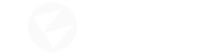# First Person Controller

Hey guys! I have a problem, when i was working in unity i created a custom fps controller and i challenged myself to do it in the flax engine so i tried to transform my unity script to flax her it is

``````using System.Collections;
using System.Collections.Generic;
using UnityEngine;

[RequireComponent(typeof(CharacterController))]

public class SC_FPSController : MonoBehaviour
{
public float walkingSpeed = 7.5f;
public float runningSpeed = 11.5f;
public float jumpSpeed = 8.0f;
public float gravity = 20.0f;
public Camera playerCamera;
public float lookSpeed = 2.0f;
public float lookXLimit = 45.0f;

CharacterController characterController;
Vector3 moveDirection = Vector3.zero;
float rotationX = 0;

[HideInInspector]
public bool canMove = true;

void Start()
{
characterController = GetComponent<CharacterController>();

// Lock cursor
Cursor.lockState = CursorLockMode.Locked;
Cursor.visible = false;
}

void Update()
{
// We are grounded, so recalculate move direction based on axes
Vector3 forward = transform.TransformDirection(Vector3.forward);
Vector3 right = transform.TransformDirection(Vector3.right);
// Press Left Shift to run
bool isRunning = Input.GetKey(KeyCode.LeftShift);
float curSpeedX = canMove ? (isRunning ? runningSpeed : walkingSpeed) * Input.GetAxis("Vertical") : 0;
float curSpeedY = canMove ? (isRunning ? runningSpeed : walkingSpeed) * Input.GetAxis("Horizontal") : 0;
float movementDirectionY = moveDirection.y;
moveDirection = (forward * curSpeedX) + (right * curSpeedY);

if (Input.GetButton("Jump") && canMove && characterController.isGrounded)
{
moveDirection.y = jumpSpeed;
}
else
{
moveDirection.y = movementDirectionY;
}

// Apply gravity. Gravity is multiplied by deltaTime twice (once here, and once below
// when the moveDirection is multiplied by deltaTime). This is because gravity should be applied
// as an acceleration (ms^-2)
if (!characterController.isGrounded)
{
moveDirection.y -= gravity * Time.deltaTime;
}

// Move the controller
characterController.Move(moveDirection * Time.deltaTime);

// Player and Camera rotation
if (canMove)
{
rotationX += -Input.GetAxis("Mouse Y") * lookSpeed;
rotationX = Mathf.Clamp(rotationX, -lookXLimit, lookXLimit);
playerCamera.transform.localRotation = Quaternion.Euler(rotationX, 0, 0);
transform.rotation *= Quaternion.Euler(0, Input.GetAxis("Mouse X") * lookSpeed, 0);
``````

after a little fine tuning i transfomed it to this

``````using System.Collections;
using System.Collections.Generic;
using FlaxEngine;

public class SC_FPSController :Script
{
public float walkingSpeed = 7.5f;
public float runningSpeed = 11.5f;
public float jumpSpeed = 8.0f;
public float gravity = 20.0f;
public Camera playerCamera;
public float lookSpeed = 2.0f;
public float lookXLimit = 45.0f;

CharacterController characterController;
Vector3 moveDirection = Vector3.Zero;
float rotationX = 0;

public bool canMove = true;

void Start()
{
characterController =  Actor as CharacterController;

// Lock cursor
CursorType. = CursorLockMode.Locked;
Cursor.visible = false;
}

void Update()
{
// We are grounded, so recalculate move direction based on axes
Vector3 forward = Actor.TransformDirection(Vector3.Forward);
Vector3 right = Transform.TransformDirection(Vector3.Right);
// Press Left Shift to run
bool isRunning = Input.GetKey(KeyboardKeys.Shift);
float curSpeedX = canMove ? (isRunning ? runningSpeed : walkingSpeed) * Input.GetAxis("Vertical") : 0;
float curSpeedY = canMove ? (isRunning ? runningSpeed : walkingSpeed) * Input.GetAxis("Horizontal") : 0;
float movementDirectionY = moveDirection.y;
moveDirection = (forward * curSpeedX) + (right * curSpeedY);

if (Input.GetAction("Jump") && canMove && characterController.IsGrounded)
{
moveDirection.Y = jumpSpeed;
}
else
{
moveDirection.Y = movementDirectionY;
}

// Apply gravity. Gravity is multiplied by deltaTime twice (once here, and once below
// when the moveDirection is multiplied by deltaTime). This is because gravity should be applied
// as an acceleration (ms^-2)
if (!characterController.IsGrounded)
{
moveDirection.Y -= gravity * Time.DeltaTime;
}

// Move the controller
characterController.Move(moveDirection * Time.DeltaTime);

// Player and Camera rotation
if (canMove)
{
rotationX += -Input.GetAxis("Mouse Y") * lookSpeed;
rotationX = Mathf.Clamp(rotationX, -lookXLimit, lookXLimit);
playerCamera.Transform.LocalRotation = Quaternion.Euler(rotationX, 0, 0);
Transform.rotation *= Quaternion.Euler(0, Input.GetAxis("Mouse X") * lookSpeed, 0);
``````

and i got some errors anyway pls help
NOTE:I know that there is an fps starter project but remember this is just a way to challenge myself

To lock and hide cursor use this:

``````Screen.CursorLock = CursorLockMode.Locked;
Screen.CursorVisible = false;
``````

To get actor forward direction you can use:

``````Actor.Transform.Forward
``````

To change camera transform and character transform use Actor properties - note that `Transform` is not Component but just a structure with Position+Rotation+Scale (Unity does it different way):

``````playerCamera.LocalOrientation = Quaternion.Euler(rotationX, 0, 0);
Actor.Orientation *= Quaternion.Euler(0, Input.GetAxis("Mouse X") * lookSpeed, 0);
``````

try out the script if it will work pls

That’s interesting. I tried a controller myself but I wanted it to be physics aware. From my experience with Unity I think you want to go either kinematic or physics-aware all the way. Mixing is asking for trouble.

Is there anybody out there who has tried a physics-aware controller?

As I wanted to have a physics-driven cart a kinematic player controller hardly can interact with it (it’s like an influence with infinite force/acceleration so it’s most probably breaking physics).
That said I had weird effects applying forces that should work but didn’t which makes me think there are unit conversion problems in Flax. If that’s the case then these should be resolved early on before people adopt that and apply forces in the magnitude of 1e9 just to make it look OK. Later on fixes will be less convenient for people who tried to balance independent on any real world values.

Well I’m currently working on that type of controller, y’all will be notified when done